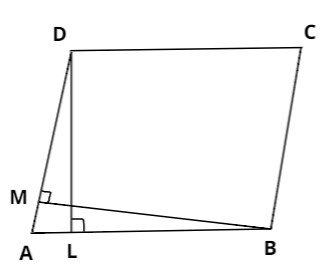Courses
Courses for Kids
Free study material
Free LIVE classes
MoreLIVE
Join Vedantu’s FREE Mastercalss

# In the given figure, $DL$ and $BM$are heights on sides $AB$ and $AD$ respectively of parallelogram $ABCD$. If the area of the parallelogram is $1470c{m^2},AB = 35cm$ and $AD = 49cm$, find the length of $BM$and $DL$.Verified
363.3k+ views
Hint: Use formula, Area of parallelogram $=$base $\times$ height.

Given, the area of parallelogram is $1470c{m^2}$and $AB = 35cm$ and $AD = 49cm$ are bases of the parallelogram.
$DL$ and $BM$ are the corresponding heights of the parallelogram.
We know that, Area of parallelogram $=$base $\times$ height. Using this formula for finding $DL$:
$\Rightarrow 1470 = AB \times DL, \\ \Rightarrow 1470 = 35 \times DL, \\ \Rightarrow DL = 42. \\$
Similarly for $BM$:
$\Rightarrow 1470 = AD \times BM, \\ \Rightarrow 1470 = 49 \times BM, \\ \Rightarrow BM = 30. \\$
Therefore, the lengths of $DL$ and $BM$ are $42cm$ and $30cm$ respectively.
Note: There is another formula of area of parallelogram as:
Area of parallelogram $= \frac{1}{2} \times$(product of diagonals).
We can use either formulae wherever required, as per our convenience.
Last updated date: 25th Sep 2023
Total views: 363.3k
Views today: 10.63k